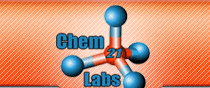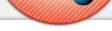Learning Pathways In General Chemistry Textbooks are filled with guides, flow-charts, approaches, patterns, pathways, etc that build and connect knowledge and facts in ways that will lead to the correct answer if followed by the learner. Unfortunately, many learners fail to accumulate this information at the appropriate time in the learning process due to poor time management skills. Assignable online exercises that require students to USE these learning pathways have been developed by Chem21Labs for both General Chemistry and Organic Chemistry. Arguably the most import concept to master in the first semester General Chemistry course is the Factor-Unit Method (sometimes referred to as Dimensional Analysis). This method of solving problems by cancelling units is presented over four to five chapters in most texts. The expectation is that the student will construct their own understanding of this process. Most textbooks present units and their inter-relationships in the following manner: Chapter 1 - English and Metric units of mass, length, area (length2) and volume are presented along with their conversion factors. Chapter 2 - cubic length (length3) is presented and its conversion factor to volume is given as 1 mL = 1 cm3. In addition, density is introduced as a conversion factor between volume and mass. Chapter 3 - moles and molecules are presented along with Avogadro's number. Also, the conversion factor of molar mass (derived from the Periodic Table) is introduced to allow the conversion between grams and moles. Chapter 4 - students are taught how to balance equations and coefficient ratios are used in Factor-Unit problems as conversion factors between moles. At this point, a student is expected to solve a complex problem like If 3.45 ft3 of Hydrogen gas is burned in excess Oxygen, what volume (in nL) of water can be produced once the water vapor condenses to produce a liquid? Density of water = 1.00 g/mL Chapter 5 - student are introduced to Molarity as the coversion factor between moles of a pure substance and Liters of a solution (usually aqueous) where the pure substance is the solute. At this point, a student is expected to solve a complex problem like If 34.5 mL of a 0.987 M NaCl is added to 30.2 mL of a 0.888 M AgNO3, how many mg of AgCl will be produced? Some students experience difficulty with the more complex questions like the ones above because they have not constructed a "personal" concept map from information presented over several weeks and chapters. These particular students benefit from pre-constructed HTML5-JS concept maps that are interactive and assignable. This "Learning Pathway" consists of clickable units that the student selects to "set up" a Dimensional Analysis problem. Next, the student must place the correct numbers in front of the units and label the units. The Map is also used to "set up" problems whose answers require two units, like density, molar mass, molar ratios or Molarity. It is important to note that "Learning Pathways" require students to answer a Dimensional Analysis problem exactly as they would find it answered in a text book, answer guide or from their instructor. By forcing students to use a method that is "universally" taught in textbooks and classrooms, the learner is supplied with a guaranteed path to success that reinforces the presentation strategies used in most textbooks and classrooms. Chem21 has also developed learning pathways for phase diagrams, heating curves, interconversion of concentration units and an extensive learning pathway for solving pH problems.